Email : info@epmstrategy.com | Call Us Now : 479-321-3977
:: Home :: Sitemap ::

# Power BI DAX HasOneValue

BY: David Rohlfs

Introduction

If you like to build large and complex DAX equations like me, then you will probably be interested in the HasOneValue () Function. This function can take a column and determine if there is only one distinct value. This is extremely helpful when you need to check your work in a DAX equation, or you just want to make sure that you only have one distinct value in an equation.

In this blog we are going to go through when to use the HasOneValue () Function and a short and simple example explaining how the function works.

When to use it

The HasOneValue () function returns a true or false for a column of a table. It determines the output on whether there is more than one distinct value in the column.

• If column A contains one instance of the product “Bike”, then the function will return True ().
• If column B contains twenty instances of the product “Handlebar”, then the function will return True ().
• If column C contains ten instances of the product “Bolt” and ten instances of the product “Hand Grip”, then the function will return False (). This is because there is more than one distinct value.

You will generally see this equation in a larger equation reviewing whether the output only contains one value. For instance, if your equation creates two values for your result, you may want to have the HasOneValue () function determine whether the equation should be reviewed or whether to add more steps to your equation.

Some of the occasions that I have found useful to use the HasOneValue () function on its own is when I am reviewing processes inside of Power BI Desktop. These are some examples:

• When setting up Row Level Security
• When checking a column only contains one distinct value
• Checking a financial report only contains one value for each day, month, year, etc.

Walkthrough

There are a few examples that I thought of for explaining how the HasOneValue () function works in Power BI, but I decided to go with an example that shows a very real-world situation. We are going to be importing an excel sheet that has flaws in it then use the HasOneValue () function to determine the correctness of the excel sheet.

To start I am going to show you this excel sheet that I built. For the purposes of this demonstration, I intentionally messed up a few of the cells.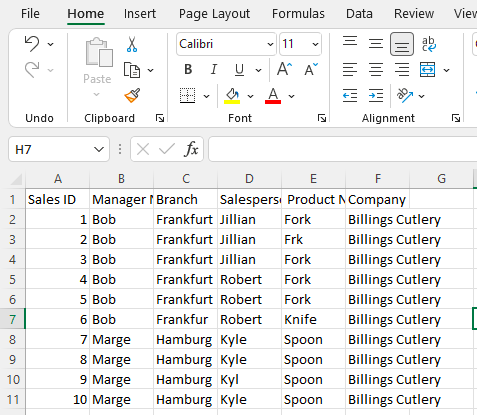I went ahead and imported this sheet into Power BI and set up this page to help us walkthrough how the function works.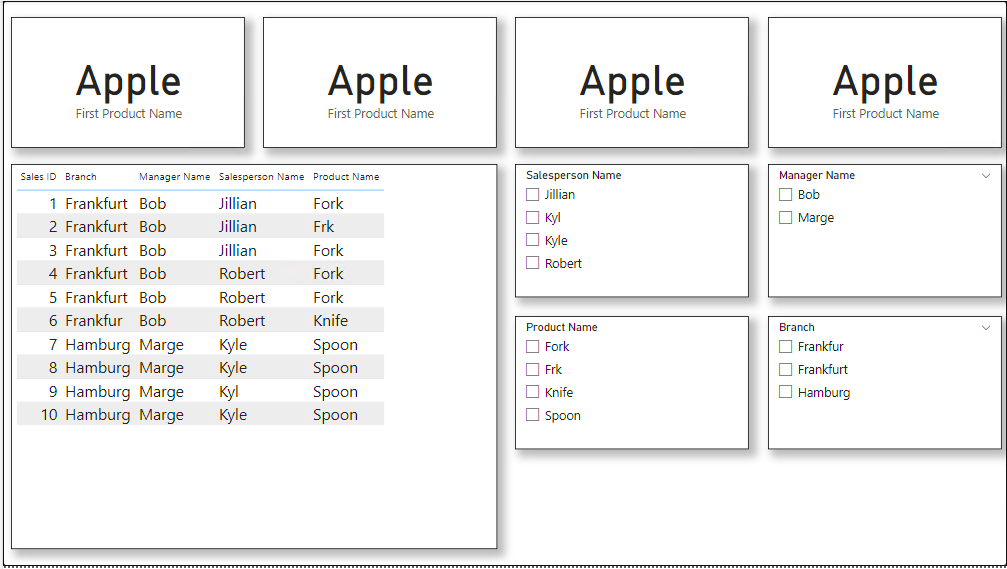I am using a measure to write out this DAX. It is a simple function that you only need to enter a table name and column name into.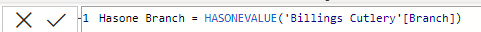I went ahead and made another three equations to check the whole sheet. This is what my page ended up looking like after I finished.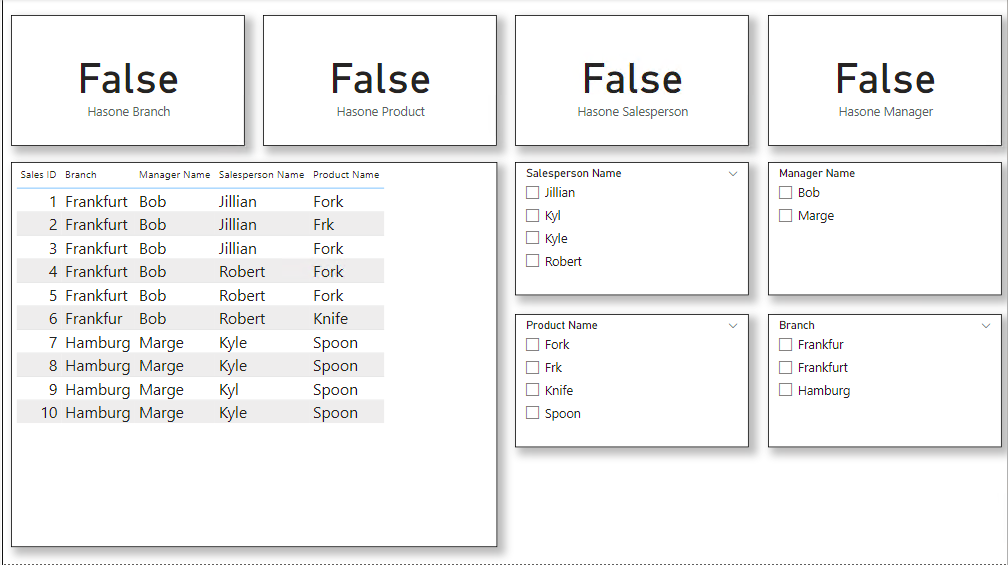You can see that everything is false right now. That is why I built the slicers so that I can cut through to the information that I know to check my work.

I wanted to Check that Jillian was only selling one product. I know that she only sells forks, so my result should be true.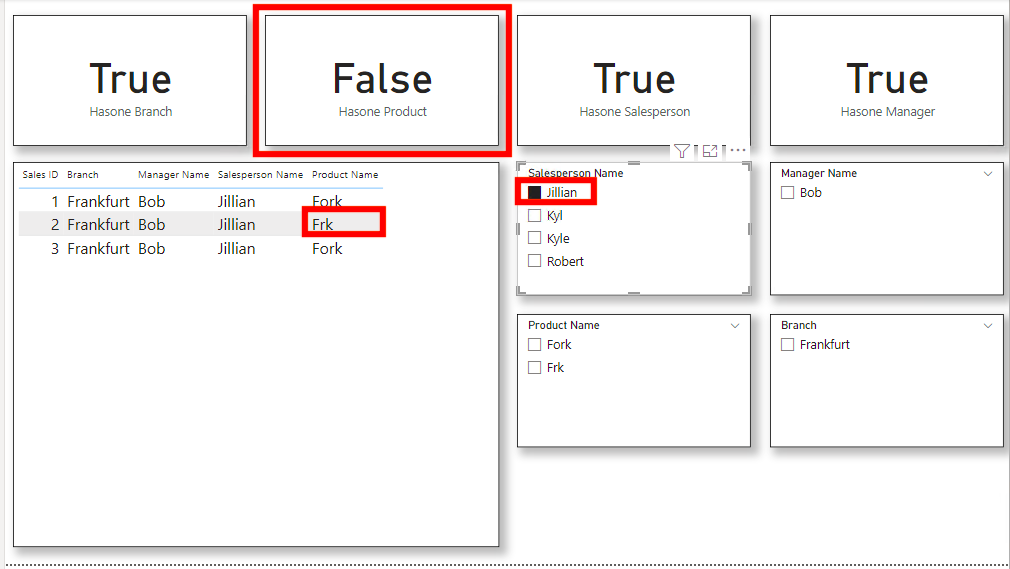In the second card at the top, the result should be true. So, I know that something inside of my dataset is wrong. There are at least two distinct values in this column. If this were a real situation I would want to go and find the incorrect data and change it so that my data would be consistent and have only one distinct value.

Although this is a lot of work for a situation like this, there is an immediate benefit to learning the basics of the HasOneValue () function. Thankfully this function can be used in much more complex situations to solve larger problems.

Conclusion

There are many other ways that you can check for only one distinct value in an equation, but this function can help a lot when you want to maximize the performance inside of your report. Unfortunately, the HasOneValue () is not great by itself unless you only want to check a distinct value, but it can be a great help inside of other equations. Hopefully this blog helps you to learn what the HasOneValue () DAX function is and the basics on what it does.

Another way to check for similar information in the power query is by using the group by function. This is through DAX and is quicker if you are only wanting to check for distinct values.

Power BI and Group BY